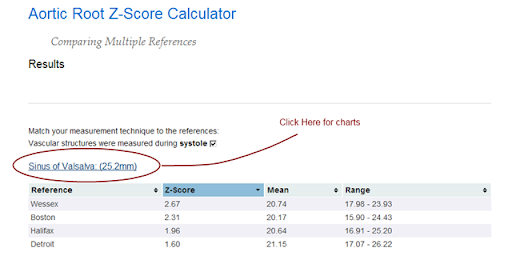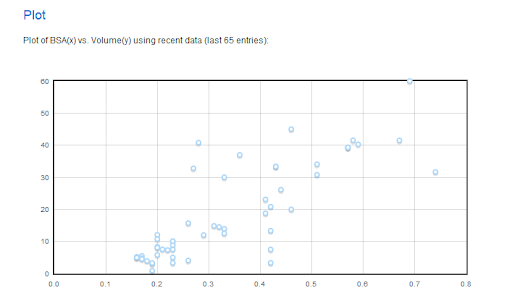## Aortic Root Reference Values Charts

A little decoration for the aortic root z-score calculator: charts.

Charts add a little ‘sugar’ to the reporting of the aortic root z-scores, by adding an important visual element to the situation.

I have tinkered with charts for the aortic root before, with a few ‘smack-down’ pages, but this is the first large-scale incorporation of the charts with the z-score calculator (well, that’s not exactly true, I do have a cute little chart for the TAPSE z-score calculator. But as far a scale , goes-- this is bigger and better.)

Now, when you see the results page from a z-score calculation, you can click on the sub-heading to link to a page with the appropriate charts for that patient:Clicking on the link illustrated in the above image takes you to these charts:

http://aoroot.parameterz.com/chart?site=sov&bsa=0.99&score=25.2

I took a small bit of design license in building these chart pages: I use the Haycock BSA formula for the patient’s BSA, even though it is not always (rarely?) the default equation used for the individual references. It made the difference between doing it in one sitting (OK, two), and probably not getting it done at all. It was just too complicated the way I had designed the base classes.

## Aortic Root and LV Volume Z-Score Calculators

Two new z-score calculators on Parameterz.com plus a paradigm shift or two

First, the z-score calculators:

1. LV End-Diastolic Volume z-scores, using the 5/6 A-L method
2. A consolidated Aortic Root z-score calculator

1. All calculations are now server-side, using Python
2. The Aortic Root calculator is a prototype for combining all available references into one calculator/results page

### LV EDV Z-Score Calculator

These z-scores are based on the recent work Normal values for left ventricular volume in infants and young children by the echocardiographic subxiphoid five-sixth area by length (bullet) method, from the Feb 2011 JASE. This is a terrific approach to what is traditionally considered a difficult measurement to calculate and normalize. For one, ventricular volumes calculated by the traditional Simpson’s bi-plane technique are notoriously unreliable. To wit, the recent abstract from the PHN citing the 5/6 A-L technique as superioir:

Variability of LV size and function measurements in children with DCM is reduced when utilizing the 5/6 AL algorithm.

(Margossian et al., JASE; May 2010)

The authors cite their own previous work as demonstrating the 5/6 A-L technique to be valid with regards to correlating well with MRI volumes.

On another front, the equations presented in this article also dramatically simplify the very complex relationship of ventricular volume and size, thought to be multi-factorial based on ejection fraction, heart rate, cardiac output, and BSA. A theoretical allometric relationship between EDV and BSA was recently described to be approximately

...the predicted relationship is EDV ≈ BSA1.4

Theoretical and empirical derivation of cardiovascular allometric relationships in children.
Sluysmans T, Colan SD.
J Appl Physiol. 2005 Aug;99(2):445-57

The calculator itself is sort-of a hybrid calculator, in that the BSA and volumes are calculated with JS on the client, then the page is posted to the server and the z-scores are calculated using Python. Another novel aspect to this calculator is that, by virtue of the calculations being performed on the server, I can provide a running tab of recent calculations, resulting in a real-time plot of actual EDV vs. BSA data:This is not the right calculator to be used for collecting normative data, but what I am learning about server-side programming-- like with this calculator-- is getting me much closer to realizing such a design.

### Aortic Root Z-Score Calculator

This calculator is a combination of what I had learned from building the LVEDV calculator and from the prototype design of the multi-referenced coronary artery z-score calculator. The essence of the design is that the patient demographics and measurement data are entered once and the z-scores and reference ranges from each of several references are returned to you in what I hope is an easy  to use, sortable, results table:

http://aoroot.parameterz.com/calc?ht=128&wt=28&aov=15.5&sov=25&stj=18&aao=21

The data required to make the calculations are part of the url (i.e., the “query string”) so the results are essentially permanent and “linkable” should anyone find any need for such a feature.

The references for calculating aortic root z-scores use various BSA equations. This calculator applies the correct BSA formula to each calculation, and thus the z-scores between each reference should be comparable, even though the calculated BSA’s are slightly different. For posterity’s sake, the different BSA calculations are also provided, which therefore makes this a very elaborate BSA calculator:

http://aoroot.parameterz.com/calc?ht=128&wt=28

Also, since not all the references use the same measurement methodology, an allowance is made to show only the appropriate calculations based on your local methodology, i.e., I filter the results based on whether the user indicates the measurement of the vascular aspects of the aortic root were measured in systole or diastole.

To be sure, all of this could have been done on the client with JavaScript, but I thought it was time to grow up.